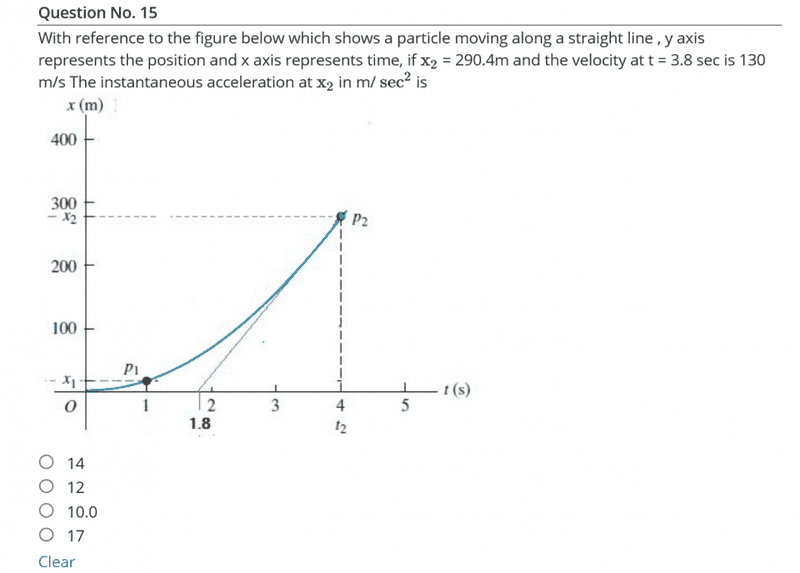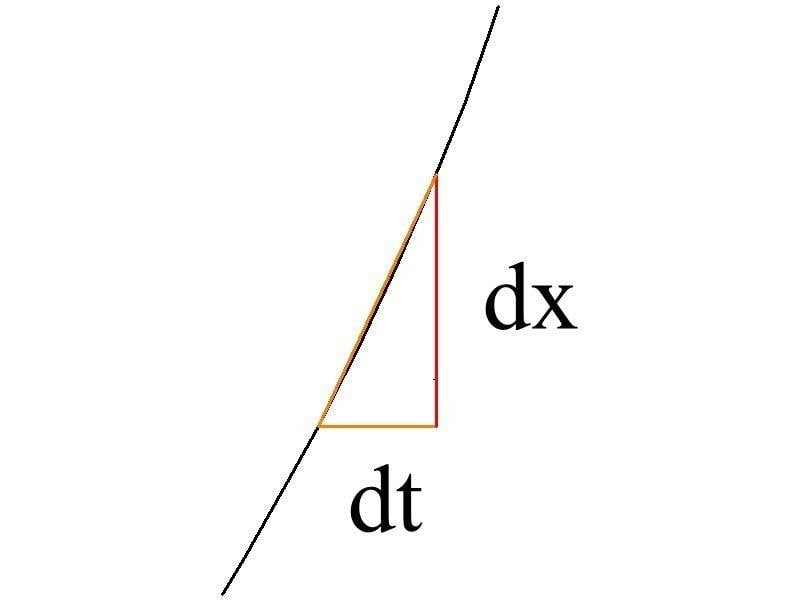# How to calculate instantaneous acceleration if -->

Member advised to use the homework template for posts in the homework sections of PF.I tried but I can't.You must show your work. What have you tried?
First I used the formula dv/dt but I am used to have a ƒ(x) in the questions I usually did.

haruspex
Homework Helper
Gold Member
First I used the formula dv/dt but I am used to have a ƒ(x) in the questions I usually did.
Write dv/dt in terms of x and y, as defined in this question.
What is the gradient at a point on a graph, a) in terms of algebra, and b) in terms of geometry?

CWatters
Homework Helper
Gold Member
+1

It looks like this question is testing that you know what dx/dt and dv/dt mean in the real world.

I am NOT getting it. Can you be a little more specific.

Nidum
Gold Member
Edit : Distorted plot corrected .

Last edited:
Nidum
Gold MemberLast edited:
•Hardikph
rcgldr
Homework Helper
There's a conflict in the figure, t2 is stated as being 3.8, but shows as 4.0 in the figure. There's insufficient information to determine instantaneous acceleration without more sample points or a limitation on the formula for acceleration versus time. If the figure was more accurate, the multiple sample points could be used to generate a function for position versus time, and then velocity and acceleration versus time. For example, the graph could be a hyperbola, in which case acceleration approaches zero as time and distance increase, or the graph could be a parabola, in which case acceleration is constant, although the graph seems to be closer to being a hyperbola.

Last edited:
•Delta2
CWatters
Homework Helper
Gold Member
There's a conflict in the figure, t2 is stated as being 3.8, but shows as 4.0 in the figure.

Sorry I disagree. I think that's deliberate.

It states the velocity at t=3.8 seconds is 130m/s and asks you to calculate the acceleration at t2=4.0 seconds. The graph gives you enough data to calculate the velocity at t2=4.0. So you have the velocities at two points a known time apart.

The accuracy might not be great but you can calculate an answer.

•Nidum
rcgldr
Homework Helper
It states the velocity at t=3.8 seconds is 130m/s and asks you to calculate the acceleration at t2=4.0 seconds. The graph gives you enough data to calculate the velocity at t2=4.0. So you have the velocities at two points a known time apart.

The accuracy might not be great but you can calculate an answer.
I wasn't paying attention to the limited choices of possible answers, so the accuracy doesn't need to be that great. There's no information after t = 4.0, so what's being calculated is the average acceleration from t = 3.8 to t = 4.0, not the instantaneous acceleration at t = 4.0. Based on the graph and the data, the acceleration is decreasing with time, instantaneous acceleration at t = 4.0 would be less.

Last edited:
haruspex